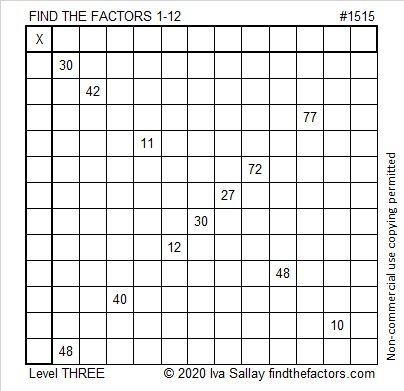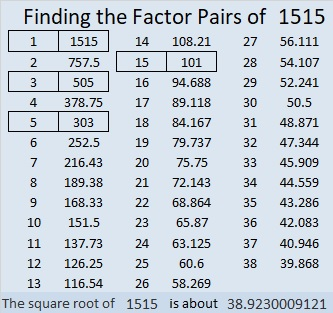# 1515 and Level 3

Contents

### Today’s Puzzle:

Start with the greatest common factor of 30 and 48, write the factors in the appropriate boxes, then work your way down this level 3 puzzle row by row using logic until you have found all the factors. You can do this!### Factors of 1515:

• 1515 is a composite number.
• Prime factorization: 1515 = 3 × 5 × 101.
• 1515 has no exponents greater than 1 in its prime factorization, so √1515 cannot be simplified.
• The exponents in the prime factorization are 1, 1, and 1. Adding one to each exponent and multiplying we get (1 + 1)(1 + 1)(1 + 1) = 2 × 2 × 2 = 8. Therefore 1515 has exactly 8 factors.
• The factors of 1515 are outlined with their factor pair partners in the graphic below.### Another Fact about the Number 1515:

1515 is the hypotenuse of FOUR Pythagorean triples:
300-1485-1515, which is 15 times (20-99-101),
651-1368-1515, which is 3 times (217-456-505),
909-1212-1515, which is (3-4-5) times 303,
1008-1131-1515, which is 3 times (336-377-505).

This site uses Akismet to reduce spam. Learn how your comment data is processed.